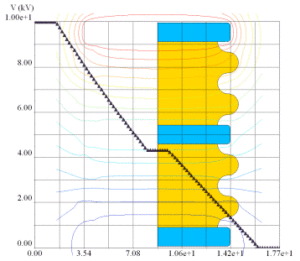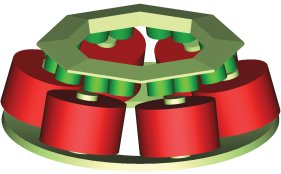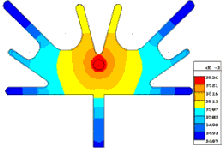# The Benefits of Having Both BEM and FEM in the Same Program

The question is often asked “Which is the better technology: Boundary Element Method or Finite Element Method?” The answer to this question depends on the problem you are solving. For some problems, BEM has huge advantages over FEM and the opposite can be said as well. The best way to look at this is to review both methods for electric, magnetic, and thermal calculations.There are a wide range of electric problems that arise. Some involve only the permittivity, others the conductivity, and for many problems both properties need to be considered in the calculation. Often for the case where the conductivity can be ignored and only the permittivity considered, we are dealing with problems where there is a large exterior region. If we consider a high voltage insulator attached to a power line, the huge open space (infinite actually) around this structure has to be included in the model. The Boundary Element Method does this naturally with no additional unknowns required. Theoretically, we can calculate the fields at infinity (zero in 3D of course) using the BEM approach. If we attempt to solve this problem using FEM we have to artificially truncate the problem by typically placing the structure in some finite box. The number of finite elements required in the space surrounding the structure can be huge. On the other hand, if we are solving a nonlinear conductive problem, where the space surrounding the conductive region is ignored, the FEM has huge advantages speed wise. Clearly for electrical problems, having both methods available can be very advantageous.

Similar situations arise for magnetic problems. Often, for problems involving magnetic sensors, there is a lot of free space around the sensor. For these applications the BEM is preferred as the free space is taken care of automatically. Also, for problems where extreme accuracy is required the BEM can often deliver where it may be almost impossible to achieve acceptable results using FEM. For highly nonlinear motor applications, where the external region is of no consequence, the FEM approach is definitely the method of choice.

For thermal applications boundary conditions must encompass the entire region. Therefore, unlike electromagnetic problems, finite elements are almost always the favored form of solution.Boundary Element analysis of an artificial heart pump – Courtesy of University of Nottingham

Now we will get down to the real benefit of having both FEM and BEM. For any real world application there is a unique solution. So independent of the method used to achieve the solution, the results should closely approximate the real world results. The question the user always has is “How good are my results?” The results of the two methods of simulation (BEM and FEM) can be compared. If they match reasonably well the user can be quite assured that the simulation data is correct. This is a very powerful method of verification of results that is of course, only available if both methods of simulation are present.

In conclusion, having FEM and BEM available in the same software has two main advantages; the right technology can be applied for the given problem and the results of both methods can be compared to assure the user that the answers are in fact correct.